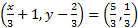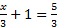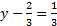# NCERT Solution for Class 11 Maths Chapter 2: Relations and Functions Exercise 2.1

In this page we have NCERT Solution for Class 11 Maths Chapter 2: Relations and Functions Exercise 2.1 . Hope you like them and do not forget to like , social share and comment at the end of the page.
Question 1
If, find the values of x and y.
GivenSince the ordered pairs are equal, the corresponding elements will also b equal.Solving these
We get,
x = 2 and y = 1
Question 2
If the set A has 3 elements and the set B = {3, 4, 5}, then find the number of elements in (A × B)?
It is given that set A has 3 elements and the elements of set B  are 3, 4, and 5.
Number of elements in set B = 3
Number of elements in (A × B)
= (Number of elements in A) × (Number of elements in B)
= 3 × 3 = 9
Thus, the number of elements in (A × B) is 9.
Question 3
If G = {7, 8} and H = {5, 4, 2}, find G × H and H × G.
G = {7, 8} and H = {5, 4, 2}
Imp Note
Cartesian product P × Q of two non-empty sets P and Q is defined as P × Q = {(p, q): p∈ P, q ∈ Q}
Therefore,
G × H = {(7, 5), (7, 4), (7, 2), (8, 5), (8, 4), (8, 2)}
H × G = {(5, 7), (5, 8), (4, 7), (4, 8), (2, 7), (2, 8)}
Question 4
State whether each of the following statement are true or false. If the statement is false, rewrite the given statement correctly.
(i) If P = {m, n} and Q = {n, m}, then P × Q = {(m, n), (n, m)}.
(ii) If A and B are non-empty sets, then A × B is a non-empty set of ordered pairs (x, y) such that x ∈ A and y ∈ B.
(iii) If A = {1, 2}, B = {3, 4}, then A × (B ∩ Φ) = Φ.
(i) False
Imp Note
Cartesian product P × Q of two non-empty sets P and Q is defined as P × Q = {(p, q): p∈ P, q ∈ Q}
If P = {m, n} and Q = {n, m}, then
P × Q = {(m, m), (m, n), (n, m), (n, n)}
(ii) True
(iii) True
Question 5
If A = {–1, 1}, find A × A × A.
It is known that for any non-empty set A, A × A × A is defined as A × A × A = {(a, b, c): a, b, c ∈ A}
It is given that A = {–1, 1}
Therefore,
A × A × A = {(–1, –1, –1), (–1, –1, 1), (–1, 1, –1), (–1, 1, 1), (1, –1, –1), (1, –1, 1), (1, 1, –1), (1, 1, 1)}
Question 6
If A × B = {(a, x), (a, y), (b, x), (b, y)}. Find A and B.
It is given that A × B = {(a, x), (a, y), (b, x), (b, y)}
It is known that Cartesian product of two non-empty sets P and Q is defined as P × Q = {(p, q): p ∈ P, q ∈ Q}
So, A is the set of all first elements and B is the set of all second elements.
Hence, A = {a, b} and B = {x, y}

Question 7
Let A = {1, 2}, B = {1, 2, 3, 4}, C = {5, 6} and D = {5, 6, 7, 8}. Verify that
(i) A × (B ∩ C) = (A × B) ∩ (A × C)
(ii) A × C is a subset of B × D
(i) To verify: A × (B ∩ C) = (A × B) ∩ (A × C)
L.H.S
We have B ∩ C = {1, 2, 3, 4} ∩ {5, 6} = Φ
Therefore,
L.H.S. = A × (B ∩ C) = A × Φ = Φ
R.H.S
A × B = {(1, 1), (1, 2), (1, 3), (1, 4), (2, 1), (2, 2), (2, 3), (2, 4)}
A × C = {(1, 5), (1, 6), (2, 5), (2, 6)}
Since the above two does not have any thing common
Therefore,
R.H.S. = (A × B) ∩ (A × C) = Φ
Hence, A × (B ∩ C) = (A × B) ∩ (A × C)
(ii) To verify: A × C is a subset of B × D
A × C = {(1, 5), (1, 6), (2, 5), (2, 6)}
A × D = {(1, 5), (1, 6), (1, 7), (1, 8), (2, 5), (2, 6), (2, 7), (2, 8),
(3, 5), (3, 6), (3, 7), (3, 8), (4, 5), (4, 6), (4, 7), (4, 8)}
We can observe that all the elements of set A × C are the elements of set
B × D. Therefore, A × C is a subset of B × D.

Question 8
Let A = {1, 2} and B = {3, 4}. Write A × B. How many subsets will A × B
have? List them.
A = {1, 2} and B = {3, 4}
It is known that Cartesian product of two non-empty sets P and Q is defined as P × Q = {(p, q): p ∈ P, q ∈ Q}
Therefore,
A × B = {(1, 3), (1, 4), (2, 3), (2, 4)}
Now   n(A × B) = 4
We know that if C is a set with n(C) = m, then Number of subsets is given by
= 2m
Therefore, the set A × B has 24 = 16 subsets.
List is given below
Φ, {(1, 3)}, {(1, 4)}, {(2, 3)}, {(2, 4)}, {(1, 3), (1, 4)}, {(1, 3), (2, 3)},
{(1, 3), (2, 4)}, {(1, 4), (2, 3)}, {(1, 4), (2, 4)}, {(2, 3), (2, 4)},
{(1, 3), (1, 4), (2, 3)}, {(1, 3), (1, 4), (2, 4)}, {(1, 3), (2, 3),
(2, 4)}, {(1, 4), (2, 3), (2, 4)}, {(1, 3), (1, 4), (2, 3), (2, 4)}

Question 9
Let A and B be two sets such that n(A) = 3 and n (B) = 2. If (x, 1), (y, 2),
(z, 1) are in A × B, find A and B, where x, y and z are distinct elements.
Given
n(A) =3 and n(B) =2; and (x, 1), (y, 2), (z, 1) are in A×B.
It is known that Cartesian product of two non-empty sets P and Q is defined as P × Q = {(p, q): p ∈ P, q ∈ Q}

Therefore,
x, y, and z are the elements of A; and 1 and 2 are the elements of B.
Now Since n(A) = 3 and n(B) = 2,
We can be defined sets as
A = {x, y, z} and B = {1, 2}.

Question 10
The Cartesian product A × A has 9 elements among which are found (–1, 0) and (0, 1). Find the set A and the remaining elements of A × A.
We know that for n(A) = p and n(B) = q, then n (A × B) = p × q.
n (A × A) = n(A) × n(A)
Given n (A × A) = 9
n(A) × n(A) = 9
n(A) = 3
The ordered pairs (–1, 0) and (0, 1) are two of the nine elements of A×A.
We know that A × A = {(a, a): a ∈ A}. Therefore, –1, 0, and 1 are elements
of A.
Since n(A) = 3, Therefore,
A = {–1, 0, 1}.
The remaining elements of set A × A are given below
(–1, –1), (–1, 1), (0, –1), (0, 0),(1, –1), (1, 0), and (1, 1).